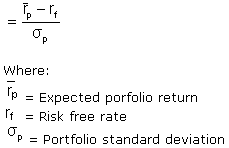# Sharpe Ratio

Financial analysis Print Email

Sharpe Ratio

Definition of Sharpe Ratio

Nobel Laureate William F. Sharpe has derived a formula that helps to measure the risk adjusted performance. As per definition, Sharpe Ratio helps in arriving at an answer which helps us analyse the risk that can be, and allowing you to make decisions on investments and also helps analyse the performance of a group.

Formula of Sharpe Ratio:(OR)Formula explained

Returns: Returns could be measured in any frequency, like daily, weekly or annually as long as the distribution of returns is normal. The major flaw of the formula lies here in the returns which can never be ascertained as normal but are indeed fluctuating.

Risk free security: Risk free security used ought to go with the period of the investment it is being compared against. You have to deduct the amount of returns from the risk free returns so that it can be divided by the standard deviation of the returns.

Standard Deviation: after calculating the excess returns by deducting risk free security and returns, we need to divide the answer with standard deviation to arrive at the answer.

An example to explain the Sharpe Ratio

Say there are two workers A and B. If A generates returns worth 30% and worker B generates returns worth 25% by looking at the returns you would analyse that A is a better worker. But if worker B has manager to take lesser risks in attaining the returns while worker A has taken major risks, the returns would be calculated otherwise.

Risk free rate is ascertained as 5% and if worker A’s standard deviation is about 7% and worker B’s is 5% then the Sharpe Ratio calculated for worker A would be 3.5 while for worker B it would be 4, which shows that worker B is a better worker and has managed a better risk-adjusted return. The higher the value of Sharpe ratio, the better it is for the business.

Although the Sharpe ratio is quite simple to use, which is one the reasons why it is so popular, there can be certain flaws that may not always be over looked. Based on three major components, the returns, the risk free returns and standard deviation the Sharpe Ratio is an easy method to find out the additional returns you can get by holding a risky asset over a risk free asset. The concept is higher the better .